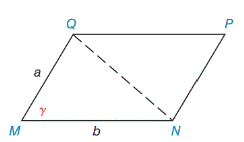Chapter 11.4, Problem 44EElementary Geometry For College St...

7th Edition
Alexander + 2 others
ISBN: 9781337614085

Solutions

Chapter
SectionElementary Geometry For College St...

7th Edition
Alexander + 2 others
ISBN: 9781337614085
Textbook Problem

The sides of a rhombus have length a. Two adjacent sides meet to form acute angle θ . Use the formula from Exercise 41 to show that the area of the rhombus is given by A = a 2  sin  θ .Exercises 41 − 44

To determine

To Prove:

The area of the rhombus is A=a2sinθ unit2.

Explanation

Given,

In rhombus,

The sides of the length is a.

(i.e.) MN=PN=PQ=QM=a

Two adjacent sides meet to from acute angle θ.

(i.e.) M=P=θ

Use the formula from exercise 41,

A=absinγ unit2.

Here, b=a and γ=θ

Still sussing out bartleby?

Check out a sample textbook solution.

See a sample solution

The Solution to Your Study Problems

Bartleby provides explanations to thousands of textbook problems written by our experts, many with advanced degrees!

Get Started

Find the limit. 44. limni=1n1n[(in)3+1]

Single Variable Calculus: Early Transcendentals

Using differentials, an approximation to (3.04)3 is: 28.094464 28.08 28.04 28

Study Guide for Stewart's Single Variable Calculus: Early Transcendentals, 8th

Evaluate 6 8 24 30

Study Guide for Stewart's Multivariable Calculus, 8th